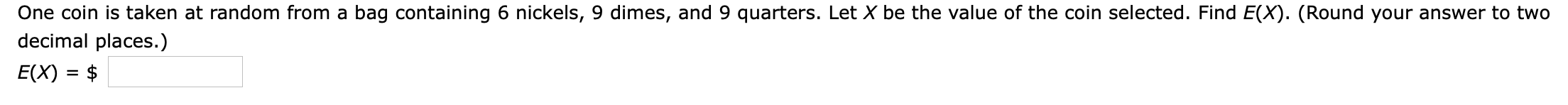# One coin is taken at random from a bag containing 6 nickels, 9 dimes, and 9 quarters. Let X be the value of the coin selected. Find E(X). (Round your answer to twodecimal places.)E(X) \$

Question
310 viewshelp_outlineImage TranscriptioncloseOne coin is taken at random from a bag containing 6 nickels, 9 dimes, and 9 quarters. Let X be the value of the coin selected. Find E(X). (Round your answer to two decimal places.) E(X) \$ fullscreen
check_circle

Step 1

There are 6 nickels, 9 dimes and 9 quarters, then total number of coins is,

Step 2

Probability of selecting a nickel is,

Step 3

Probability of select...

### Want to see the full answer?

See Solution

#### Want to see this answer and more?

Solutions are written by subject experts who are available 24/7. Questions are typically answered within 1 hour.*

See Solution
*Response times may vary by subject and question.
Tagged in

### Math